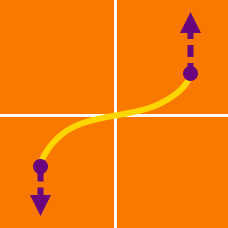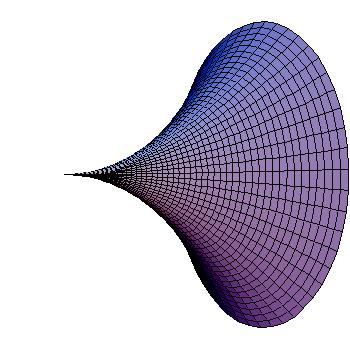Calculus

# Arc Length and Surface Area: Level 3 Challenges

Lise jogs along the curve defined by $f(x) =\dfrac{2(x - 1)^{\frac{3}{2}}}{3}$ from $(1, f(1))$ to $(4, f(4)).$ Steve jogs along the straight line connecting those two points. Steve and Lise both start from $x = 1$ at the same time and Lise jogs at a speed of $\frac{7}{\sqrt{3}} \mbox{ units}\mbox{/s}$. What is the speed at which Steve must run (in $\mbox{ units}\mbox{/s}$) so that he arrives at $(4, f(4))$ at the same time as Lise?

The coordinates of a dot $P$ moving in the $xy$ plane at time $t$ are given by: $x = 5e^{-t}\cos t$ and $y = 5e^{-t} \sin t$. Let $L_a$ be the distance traveled by $P$ in the interval $0 \leq t \leq a$. If $\displaystyle \lim_{a \to \infty} L_a = z$, what is the value of $z^2$?

A delivery drone flying at constant speed $15 \text{ m/s}$ and constant height $2700 \text{ m}$ toward a destination drops its goods. If the trajectory of the falling goods until it hits the ground can be described by the equation $y=2700-\frac{x^2}{75},$ where $x$ is the horizontal distance it travels and $y$ is its height above the ground, what is the distance (not horizontal displacement) traveled by the goods until it hits the ground?

Note: You can use $\displaystyle \int \sqrt{a^2+u^2}\,du=\frac{u}{2}\sqrt{a^2+u^2}+\frac{a^2}{2}\ln(u+\sqrt{a^2+u^2})+C.$There exists a unique, positive-valued, non-constant, continuous and differentiable function $y = f(x)$ such that

(i) over any specified interval, the area between $f(x)$ and the $x$-axis is equal to the arclength of the curve, and

(ii) $f(0) = 1$.

If $S = \displaystyle\int_{-1}^{2} f(x) dx$, then find $\lfloor 1000S \rfloor$.Consider the curve $y={ e }^{ -x }$ in the first quadrant. Now it's rotated about the $x\text{-axis}$ to obtain a solid of revolution. What is its surface area to 4 decimal places?

×